## Graphics extensions

This pages lists the graphics extensions available in the ARM and RISC-V versions of uLisp.

They currently support the displays on the following boards:

These extensions are based on and compatible with Adafruit's GFX library .

For examples of using these routines see Graphics examples.

### Summary

#### Colours

Most of the plotting functions take a colour parameter to specify the colour of plotting. This is a 16-bit number specifying the red, blue, and green components of the colour: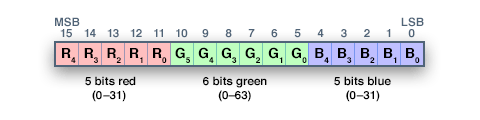The following function rgb allows you to define a colour by specifying the red, green, and blue components as numbers between 0 and 255:

```(defun rgb (r g b)
(logior (ash (logand r #xf8) 8) (ash (logand g #xfc) 3) (ash b -3)))```

For example, to define an orange colour you could write:

`(defvar orange (rgb 255 127 0))`

### draw-char function

Syntax: `(draw-char x y char [colour background size])`

Draws the character char with its top left corner at (x, y). The character is drawn in a 5 x 7 pixel font in colour against background, which default to white and black respectively. The character can optionally be scaled by size.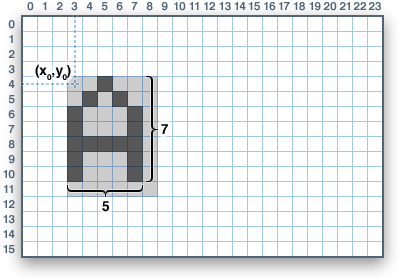#### Built-in character set

Text plotting uses a built-in character set which is based on the IBM PC Code Page 437 character set: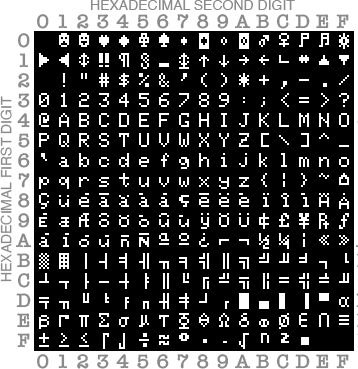### draw-circle function

Syntax: `(draw-circle x y r [colour])`

Draws an outline circle with its centre at (x, y) and with radius r. The outline is drawn in colour, or white if omitted.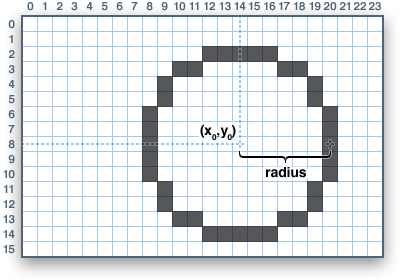### draw-line function

Syntax: `(draw-line x0 y0 x1 y1 [colour])`

Draws a line from (x0 , y0) to (x1, y1) in colour, or white if omitted.

### draw-pixel function

Syntax: `(draw-pixel x y [colour])`

Draws a pixel at coordinates (x , y) in colour, or white if omitted.

### draw-rect function

Syntax: `(draw-rect x y w h [colour])`

Draws an outline rectangle with its top left corner at (x, y), with width w, and with height h. The outline is drawn in colour, or white if omitted.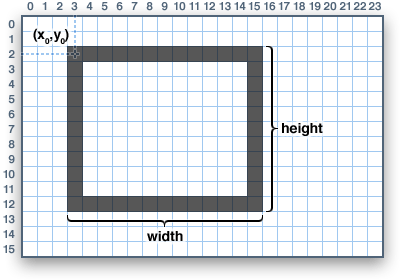### draw-round-rect function

Syntax: `(draw-round-rect x y w h radius [colour])`

Draws an outline rounded rectangle with its top left corner at (x, y), with width w, height h, and corner radius radius. The outline is drawn in colour, or white if omitted.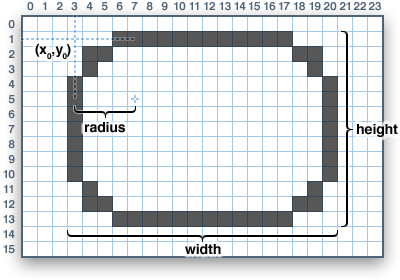### draw-triangle function

Syntax: `(draw-triangle x0 y0 x1 y1 x2 y2 [colour])`

Draws an outline triangle between (x1, y1), (x2, y2), and (x3, y3). The outline is drawn in colour, or white if omitted.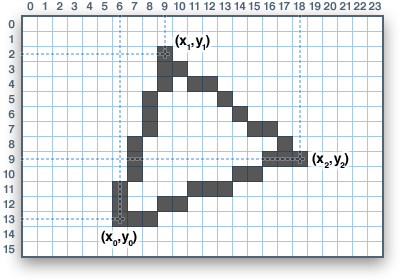### fill-circle function

Syntax: `(fill-circle x y r [colour])`

Draws a filled circle with its centre at (x, y) and with radius r. The circle is drawn in colour, or white if omitted.

### fill-rect function

Syntax: `(fill-rect x y w h [colour])`

Draws a filled rectangle with its top left corner at (x, y), with width w, and with height h. The rectangle is drawn in colour, or white if omitted.

### fill-round-rect function

Syntax: `(fill-round-rect x y w h radius [colour])`

Draws a filled rounded rectangle with its top left corner at (x, y), with width w, height h, and corner radius radius. The frame is drawn in colour, or white if omitted.

### fill-screen function

Syntax: `(fill-screen [colour])`

Fills or clears the screen with colour, default black.

### fill-triangle function

Syntax: `(fill-triangle x0 y0 x1 y1 x2 y2 [colour])`

Draws a filled triangle between (x1, y1), (x2, y2), and (x3, y3). The triangle is drawn in colour, or white if omitted.

### invert-display function

Syntax: `(invert-display boolean)`

Mirror-images the display.

### set-cursor function

Syntax: `(set-cursor x y)`

Sets the start point for text plotting to (x, y).

### set-text-color function

Syntax: `(set-text-color colour [background])`

Sets the text colour for text plotted using with-gfx.

### set-text-size function

Syntax: `(set-text-size scale)`

Scales text by the specified size, default 1.

### set-text-wrap function

Syntax: `(set-text-wrap boolean)`

Specified whether text wraps at the right-hand edge of the display; the default is t.

### set-rotation function

Syntax: `(set-rotation option)`

Sets the display orientation for subsequent graphics commands; values are 0, 1, 2, or 3.

### with-gfx special form

Syntax: `(with-gfx (stream) form*)`

The with-gfx form evaluates the forms with stream bound to a gfx-stream.

You can then print text to the graphics display using the standard uLisp print commands. To control printing use set-cursor, set-text-color, set-text-size, and set-text-wrap.

For example, the following function pretty-prints the currently defined functions to the display:

```(defun gprint ()
(fill-screen)
(set-rotation 2)
(with-gfx (str)
(set-cursor 0 0)
(pprintall str)))```

1. ^ Diagrams reproduced from Adafruit under the Attribution-ShareAlike Creative Commons licence.